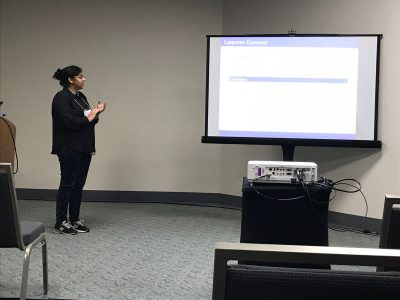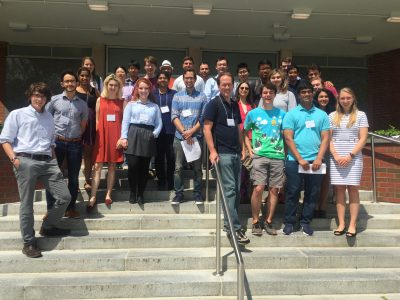Hedging by Sequential Regression in Generalized Discrete Models and the Follmer-Schweizer decompositionOverview

In practice, financial models are not exact — as in any field, modeling based on real data introduces some degree of error. However, we must consider the effect error has on the calculations and assumptions we make on the model.  In complete markets, optimal hedging strategies can be found for derivative securities; for example, the recursive hedging formula introduced in Steven Shreve’s “Stochastic Calculus for Finance I” gives an exact expression in the binomial asset model, and as a result the unique arbitrage-free price can be computed at any time for any derivative security.

In incomplete markets this cannot be accomplished; one possibility for computing optimal hedging strategies is the method of sequential regression.  We considered this in discrete-time; in the (complete) binomial model we showed that the strategy of sequential regression introduced by Follmer and Schweizer  is equivalent to Shreve’s recursive hedging formula, and in the (incomplete) trinomial model we both explicitly computed the optimal hedging strategy predicted by the Follmer-Schweizer decomposition and we showed that the strategy is stable under small perturbations.

forthcoming

arXiv:1808.03186

Ayelet Amiran, Fabrice Baudoin, Skylyn Brock, Berend Coster, Ryan Craver, Ugonna Ezeaka, Phanuel Mariano, Mary Wishart

project page:

Math UConn REU at JMM 2018

Two of our REU (2017 Stochastics) participants, Raji Majumdar and Anthony Sisti, will be presenting posters Applications of Multiplicative LLN and CLT for Random Matrices and Black Scholes using the Central Limit Theorem on Friday, January 12 at the MAA Student Poster Session, and both of them will be giving talks on Saturday, January 13 at the AMS Contributed Paper Session on Research in Applied Mathematics by Undergraduate and Post-Baccalaureate Students.

Their travel to the 2018 JMM has been made possible with the support of the MAA and UConn’s OUR travel grants.Financial Math: Portfolio Optimization and Dynamic Programming

Group Members

Ayelet Amiran, Skylyn Brock, Ryan Craver, Ugonna Ezeaka, Mary WishartOverview

Financial markets have asymmetry of information when it comes to the prices of assets. Some investors have more information about the future prices of assets at some terminal time. However, what is the value of this extra information?

We studied this anticipation in various models of markets in discrete time and found (with proof) the value of this information in general complete and incomplete markets. For special utility functions, which represent a person’s satisfaction, we calculated this information for both binomial (complete) and trinomial (incomplete) models.

arXiv:1808.03186

tba

Poster

PosterFinMath2018

5th Northeast Mathematics Undergraduate Research Mini-Symposium

University of Connecticut, August 3rd, 2017

Participating Schools: Amherst, Smith, UConn and UMass

5th Mini-Symposium full program (2017)Multiplicative LLN and CLT and their Applications

Overview

We study the Law of Large Numbers (LLN) and and Central Limit Theorems (CLT) for products of random matrices. The limit of the multiplicative LLN is called the Lyapunov exponent. We perturb the random matrices with a parameter and we look to find the dependence of the the Lyapunov exponent on this parameter. We also study the variance related to the multiplicative CLT. We prove and conjecture asymptotics of various parameter dependent plots.

Presentations:

Raji Majumdar and Anthony Sisti, will present posters Applications of Multiplicative LLN and CLT for Random Matrices and Black Scholes using the Central Limit Theorem on Friday, January 12 at the MAA Student Poster Session, and give talks on Saturday, January 13 at the AMS Contributed Paper Session on Research in Applied Mathematics by Undergraduate and Post-Baccalaureate Students.Stochastic Stability of Planar Flows

Overview

We investigated systems of complex-valued ordinary differential equations (ODEs) that blows up in finite time, which we refer to as explosive systems. The goal is to understand for what initial conditions does the system explode and will the addition of noise stabilize it; that is, if we were to perturb the system with an additive Brownian motion, will the system of stochastic differential equation (SDE) still be explosive? In fact, we were able to prove a toy model of the stochastic Burgers’ equation to be ergodic; that is, the SDE is nonexplosive and it has a unique limiting distribution.

Poster